﻿ Collect Forms – Formulas

# Collect Forms – Formulas

Formulas add the ability to narrow results, and to select, view, and automate different types of fields and parameters. The Formula Builder is used to create complex formulas that can be configured using the available functions, standard fields, section fields, meta fields, and aggregate fields from selected forms. These options are available in the Formula Object Editor.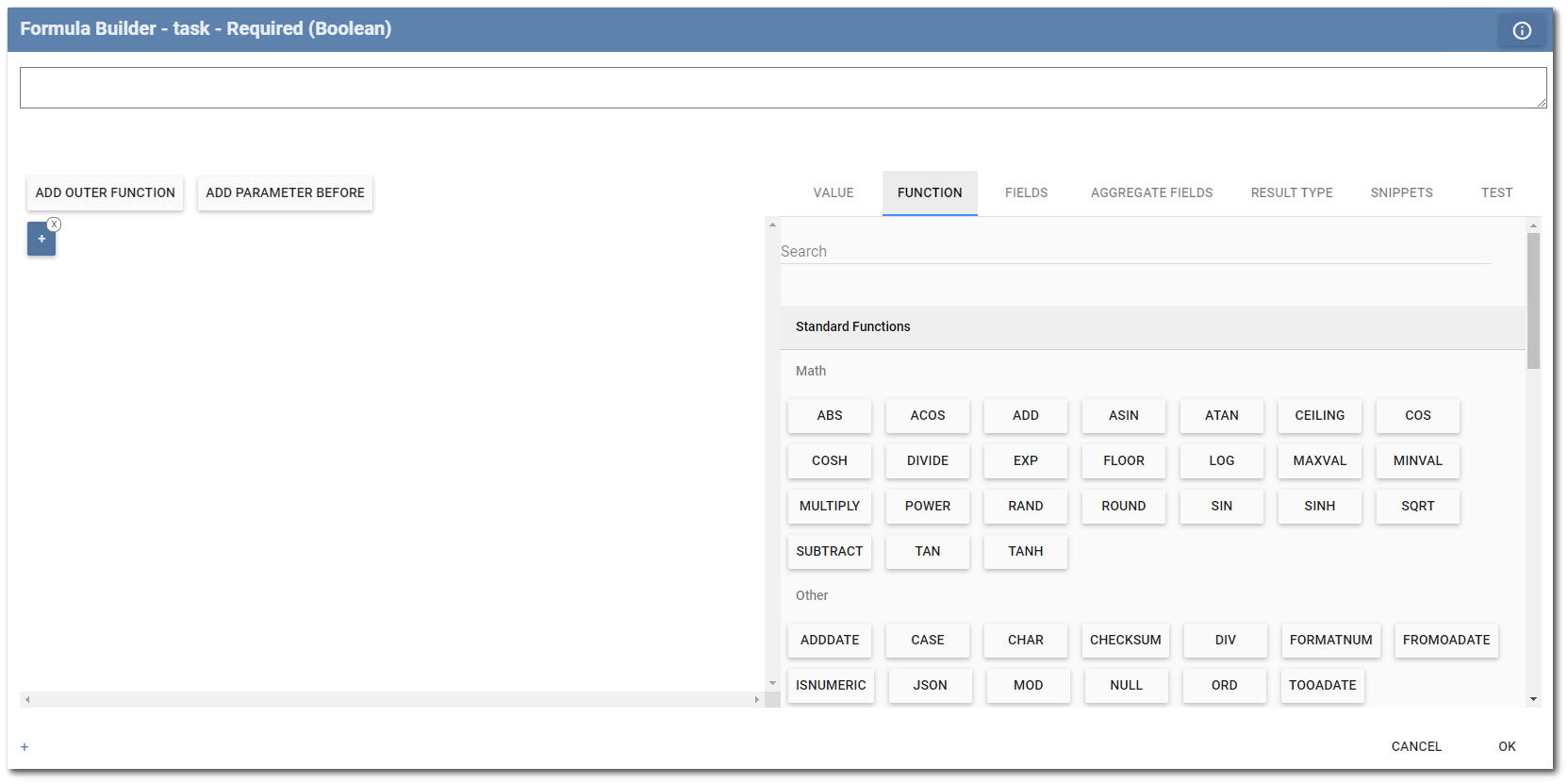Value – The actual value attributed to a field. Values may be numeric (start with a number) or a string (start with a quote). Value Type radio buttons allow a user to set the type of node as "Text" or "Number", giving users additional control over how a formula evaluates in a function. For example, if a user enters a value of '00009' and selects a Text Value Type, the value would remain '00009', but if the Value Type was changed to Number, the value would become 9. Some field values, such as a geography field, have string representations that are in JSON (JavaScript Object Notation) format which uses the following syntax: {"key_1":"value_1", "key_2":"value_2",..."key_n":"value_n"}. To enter an empty/blank value, select the Empty check box on the right-hand side and formula parser will add an empty string value of '' to the formula.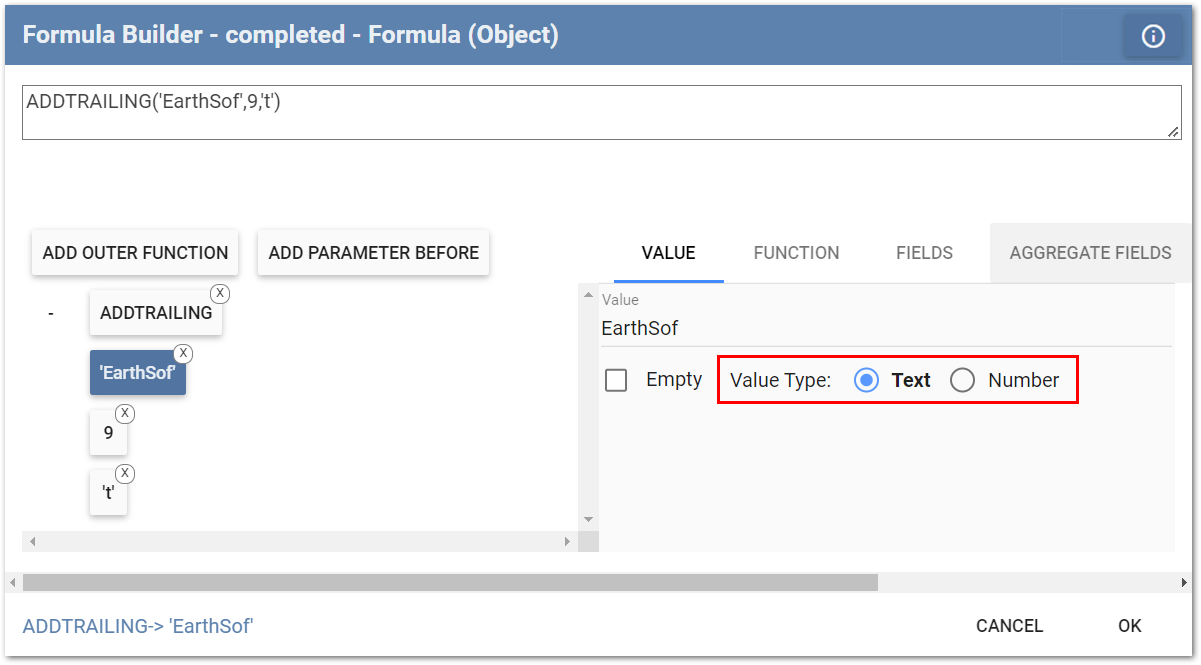Function – This tab provides a list of all functions available to use in building formulas. Hovering the mouse over any function provides a tooltip summary of the function. The functions are described in detail in the Functions chapter and examples are provided.

Fields – This tab provides access to form fields on the current form or a parent form as well as meta data. Fields are grouped by Standard Fields and Section Fields. Standard Fields are fields directly from the form (current or parent). Section Fields are general, universal field values available for all fields on the form. See Section Fields for more information. Meta fields are fields associated to the entire form template and have values that do not change from form to form. See Meta Fields for more information.Aggregate Fields – Refers to fields inside aggregate functions, which process all values of fields on specific tables. Aggregate functions and fields allow the extraction of data from other tables and executing more complex validation tasks. For example, a user can determine if a measured value is stable (has not changed much over the last three readings) or can extract a value from a related form.

Result Type – When using a formula field, this tab allows the user to set the result type that the formula will return. Setting the result type will force attribute values to be converted to the corresponding type.

For example, if the result type is set as a decimal, the number of digits after the decimal can be specified using the Set Scale attribute. The attribute result will round the value to the corresponding number of digits when saved or used in other formulas. For example, a formula field that multiplies two other numeric fields together can be defined to round to two decimal places.

A "Format" option is available for result types other than String (i.e., a collection of characters within quotation marks, such as "example of a string"), Object (i.e., an object is created from a class which is like a blueprint for creating objects), or Boolean (i.e., data type that can only be TRUE or FALSE). This option uses the Microsoft dotNet format options for the given result type. When a format is specified, then the result of the formula will always be a string. The formula result value will be converted to the specified type and then converted to the string representation using the provided format string.

Snippets – This tab allows the user to save a formula to use in another field or on other forms, described in detail in the Formula Snippets article.

Test – This tab allows for testing various pieces of a formula. With the Test tab active, a user can:

1.Select a field name from the Formula Tree View and enter a Test Value.

2.Select any function from the Formula Tree View to see how it and its parameter(s) evaluate.

 Notes: •Test Values will not be accepted for aggregate fields. To fully test formulas, including those using aggregate fields, set the template to Test status, download it to Collect Mobile, and enter the data in the form(s) needed to test the formula. •Fields with Default attribute values are required to be visible for the formula to properly function. For formula(s) to work with a hidden field(s), the field(s) must be a formula or pre-populated field.

A Search bar is available in the function, field, and aggregate field tabs. The Search bar allows users to quickly and easily search for specific functions and form fields.

## Fields Tab Example

The fields shown in the Fields tab in the Formula Object Editor of the Formula Builder correspond to fields added to the form template, as designed on the Template Designer page. The fields are organized by the forms to which they belong. In this example, the Boring Log template has two parent forms: Project and Borehole. The Borehole form is a parent form with two child forms.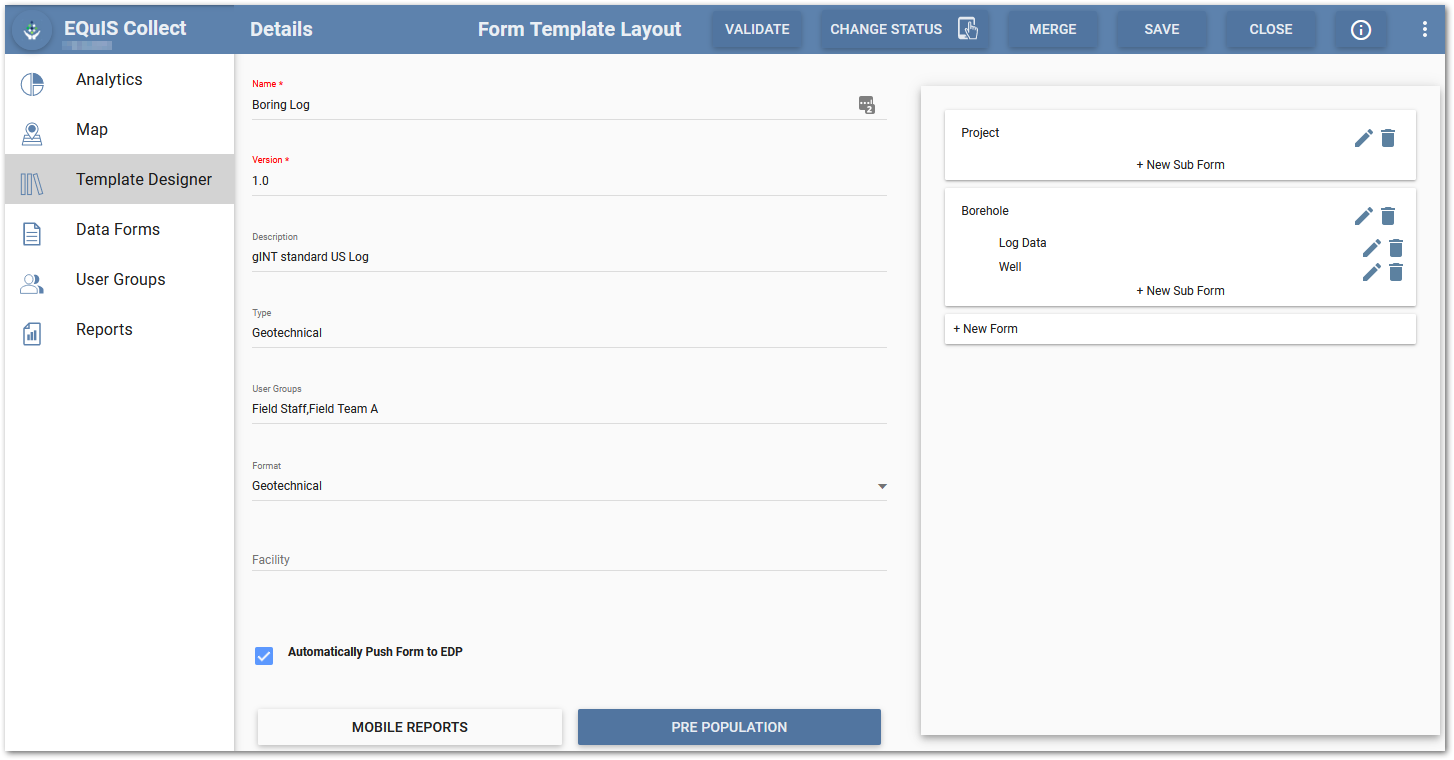The image below shows the Borehole form and its associated fields.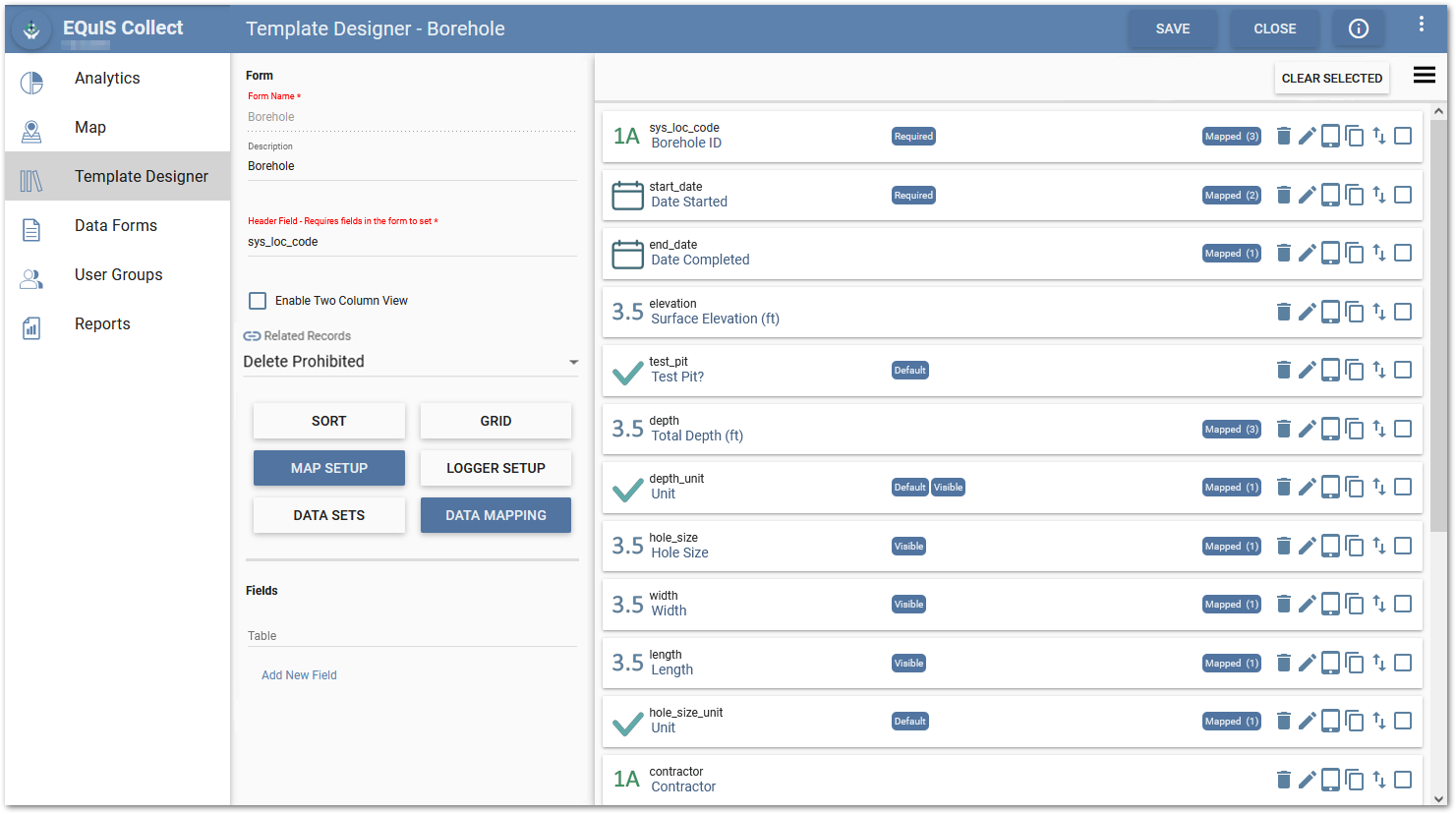The Fields tab displays the standard fields and section fields (i.e., system fields) that belong to the form currently being configured. In this example, the fields relate to the Boring form and its associated child forms.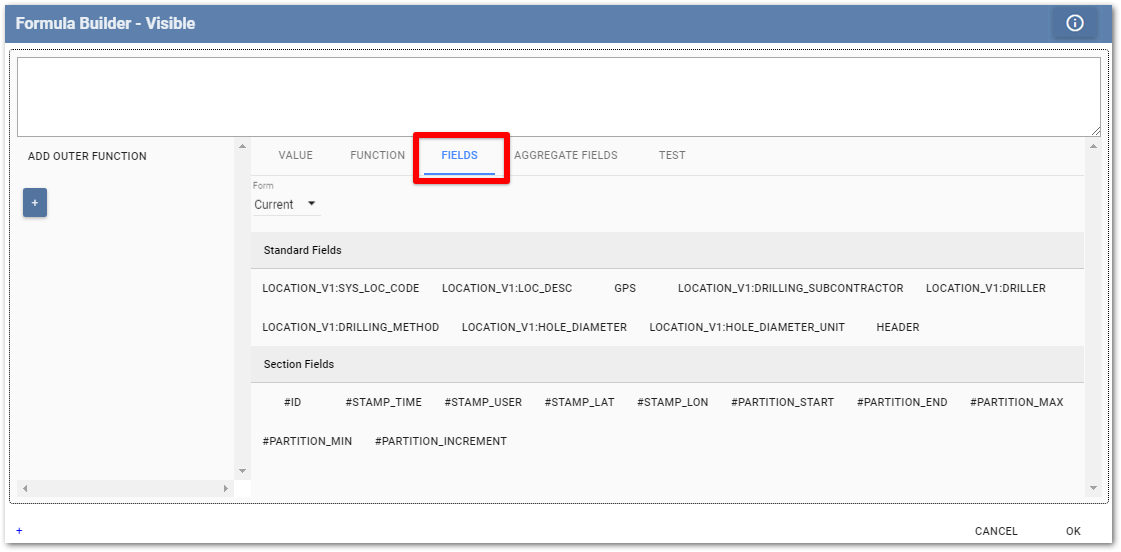While still in the Formula Builder, fields from the current form and all other forms in the template can be accessed by selecting the Aggregate Fields tab.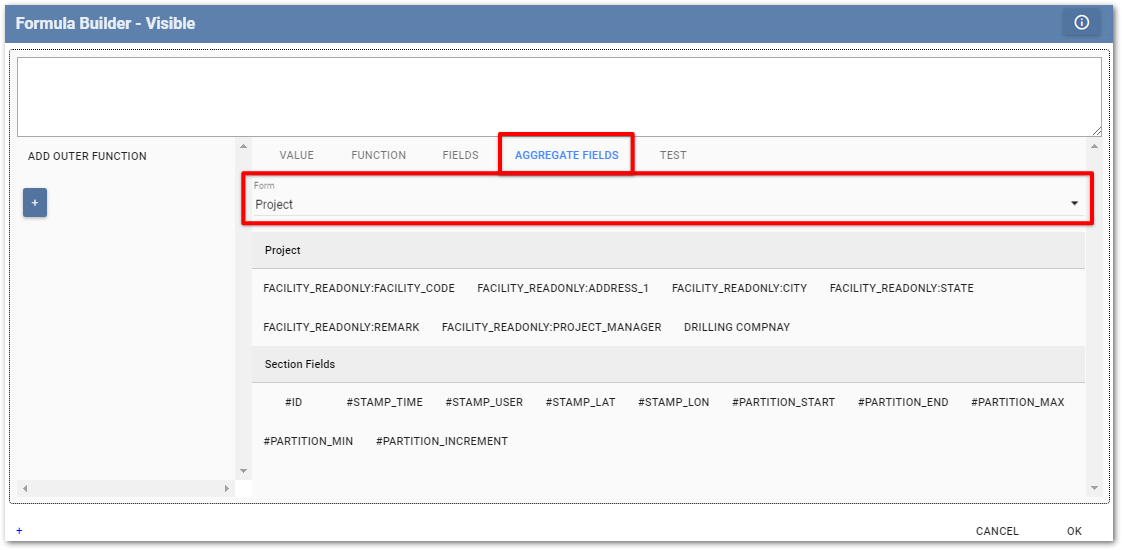## How to Build a Formula

A new formula can be created by typing directly into the Formula Text Editor pane or can be built by clicking on the + button in the Formula Tree View to add a formula component from the Formula Object Editor pane. Choose what type of formula to build by selecting the desired component tab in the Formula Object Editor pane (e.g., Functions).

The Search String box in the Formula Object Editor pane can be used to filter the functions or fields to easily find the desired item. Search help is also provided as the user types in the Formula Text Editor pane. Possible matches appear for selection with a brief description to understand the function.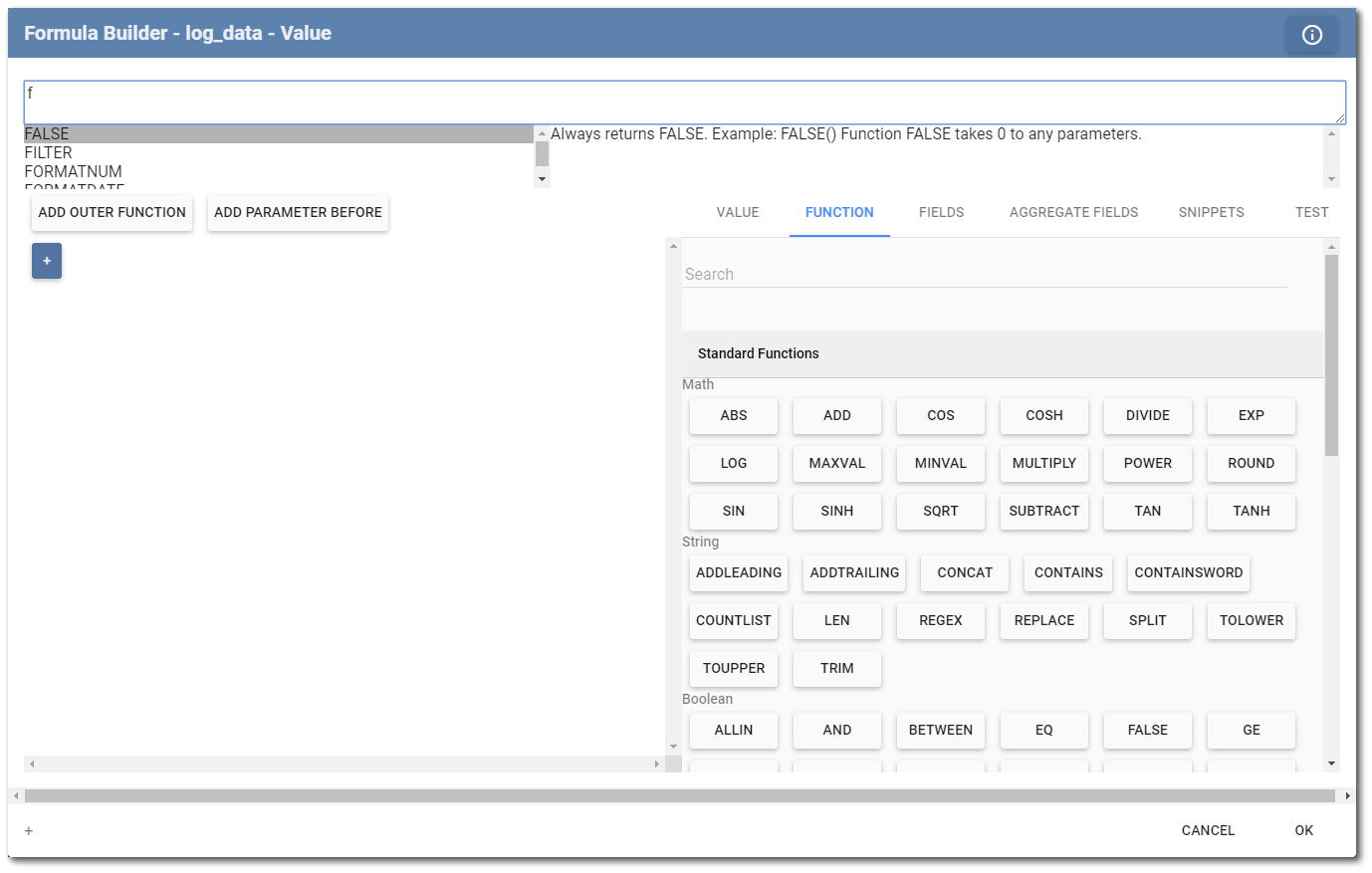The Add Outer Function and Add Parameter Before buttons can be used to help construct the formula in the Formula Tree View. As formula components are added, they appear in both the tree view and the text editor pane.

To delete a component, either (1) click thesymbol in the upper right corner of the component in the tree view or (2) delete the text in the text editor pane and hit the Enter key.

The image below illustrates a constructed formula.Using text formulas to calculate values involves two distinct processes: Parsing and Evaluating.

Parsing – Process of interpreting the text and converting it into a set of equivalent computer language objects called a parse tree. Pressing the Enter key while in the formula text editor runs the parser.

Evaluating – Process of using the parse tree objects to return a specific value based on the values of the field objects in that tree. The Test tab in the Formula Object Editor pane allows for evaluating various pieces of a formula by entering test values.

## Formula Syntax

The formula parser interprets formula text strings using a recursive algorithm that matches the formula text syntax. This is beneficial since it increases parsing performance while simplifying the formula writing syntax and the code required to parse. To capture these benefits, users may experience a small learning curve when first writing formulas.

The formula syntax is such that at any level (the trunk or branch of the parse tree) a formula is simply a single parameter.  A parameter, however, can be of three distinct types: A constant, a field, or a function. Each of these parameter types has specific valid starting characters that allow the parser to interpret them as such and “parse” them into their corresponding object types.

Constants – Can be either fixed decimal numeric values that must start with a numeric digit, or string constants that must be enclosed with single quotes. Single quotes will be applied automatically if a string value is entered directly into the value tab of the formula builder.

Fields – Can be any field name enclosed in square brackets with or without a table name prefix (e.g., [table.field]). Square brackets will be applied automatically if a field is selected directly from fields tab of the formula builder. It should be noted that table name prefixes are required when the field values need to change based on the rows in a table (e.g., when using aggregate functions). If the table name prefix is not added to the field, then the field value is fixed at the value of the current row.

Functions – Can be any valid function name that must start with a letter or underscore and must end with a round parentheses that contains the parameter(s) used in the function. For example, FUNCTIONNAME(param1,param2,param3) defines a function called FUNCTIONNAME with three parameters.

Since functions can contain parameters (any parameters) within their parentheses, any calculation can be achieved based on these three general parameter types with no ambiguity as to order of precedence (i.e., the order in which operations are to be executed).

### Example

If a user wanted to multiply two values (3 and 5) together, the formula syntax would be:

MULTIPLY(3,5), which would return a value of 15.

To add the value of field X to 5, the formula syntax would be:

MULTIPLY(3,SUM(5,[X]))

In this case, the first parameter of the MULTIPLY function is a constant value of 3 and the second parameter is a SUM function with two parameters (constant parameter 5 and field parameter X).